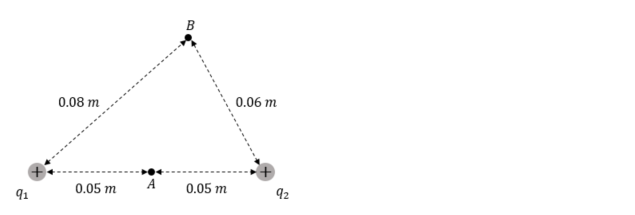# Problem: Two point charges q1 = +10.00 nC and q 2 = +2.00 nC are 0.100 m apart as shown in the figure to the right. Point A is midway between q1 and q2. Point B is 0.080 m from q 1 and 0.060 from q 2. Determine the energy required to move a charge q 3 = +10.00 nC from point B to point A.

###### FREE Expert Solution
93% (388 ratings)
###### Problem Details

Two point charges q1 = +10.00 nC and q 2 = +2.00 nC are 0.100 m apart as shown in the figure to the right. Point A is midway between q1 and q2. Point B is 0.080 m from q 1 and 0.060 from q 2. Determine the energy required to move a charge q 3 = +10.00 nC from point B to point A.Frequently Asked Questions

What scientific concept do you need to know in order to solve this problem?

Our tutors have indicated that to solve this problem you will need to apply the Work From Electric Force concept. You can view video lessons to learn Work From Electric Force. Or if you need more Work From Electric Force practice, you can also practice Work From Electric Force practice problems.

How long does this problem take to solve?

Our expert Physics tutor, Juan took 5 minutes and 47 seconds to solve this problem. You can follow their steps in the video explanation above.

What professor is this problem relevant for?

Based on our data, we think this problem is relevant for Professor Hummel's class at UIUC.